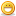1. ## Simple one

In the five digit number 7a1a4 each of a's represent a same digit and if this number is divisible by 9 then a stands for...?Reply With Quote

2. ## Re: Simple one

ans is a=3

to solve it easily

remember a number is divisible by 9 if the sumof its digits is divisible by 9.

here number is

7a1a4

7+1+4=12

12+2a should be equal to 18

2a=6
therefore a=3byeReply With Quote

3. ## Re: Simple oneReply With Quote

4. ## Re: Simple one

HI,

Regards

Nikhil RattanReply With Quote

5. ## Re: Simple one

Yeps. The answer is right. a=3. Just substitute the value and divide y 9, you'll see that it is divisible by 9.

73134/9 = 8126.

-KalayamaReply With Quote

6. ## Re: Simple oneReply With Quote

7.

####Posting Permissions

• You may not post new threads
• You may not post replies
• You may not post attachments
• You may not edit your posts
•